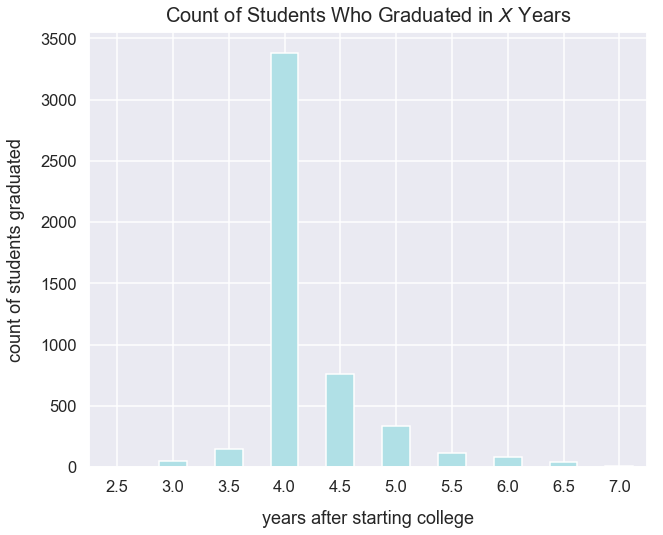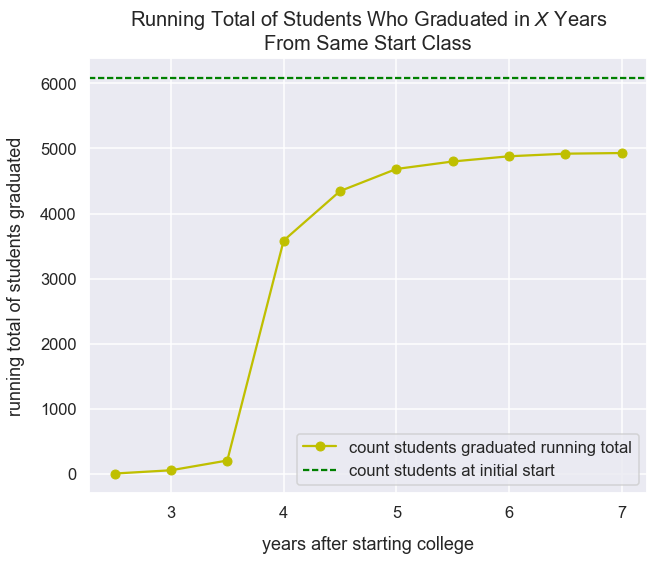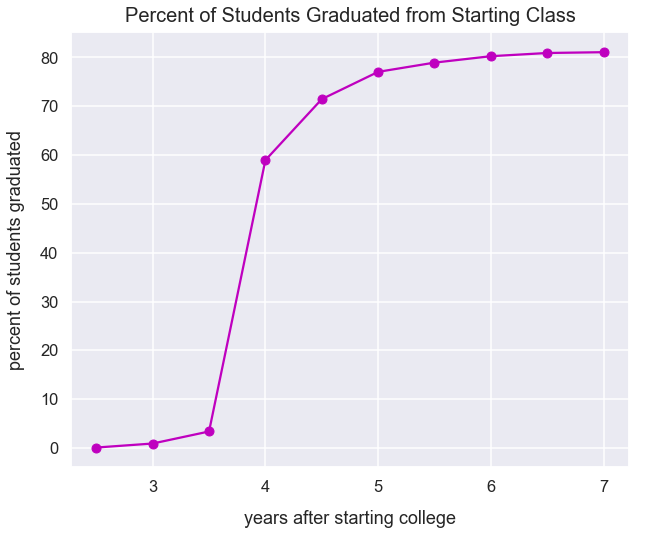Data Visualizations Best Practices Tutorial

# When to Use a Cumulative Frequency Graph

#### Import Modules¶

In :
import pandas as pd
import matplotlib.pyplot as plt
import seaborn as sns
% matplotlib inline


#### Set Visualization Styles¶

In :
sns.set_style("darkgrid")
sns.set_context("talk")


I generate fictional data for the count of students who graduated college in years after having the same start date.

I assign the variable count_students_at_class_start as the total number of students who started college at the same time, yet not all these students graduated.

In :
count_students_at_class_start = 6080

graduation_data = {'years_after_starting_college': [2.5, 3, 3.5, 4, 4.5, 5, 5.5, 6, 6.5, 7],
'count_students_graduated': [5, 50, 150, 3380, 760, 340, 115, 80, 40, 10]}


Preview the data in df.

In :
df.head()

Out:
0 2.5 5
1 3.0 50
2 3.5 150
3 4.0 3380
4 4.5 760

Initially, we can plot this data as a bar graph for the count of students who graduated in each half-year interval.

In :
df.plot(kind='bar', x='years_after_starting_college', y='count_students_graduated',
figsize=(10, 8), legend=False, color='powderblue', rot=0);
plt.title("Count of Students Who Graduated in $X$ Years", y=1.01, fontsize=20)I find this visualization a bit confusing. With each bar, it's tough to understand the significance. So almost 3,000 students graduated in 4 years...is that good? How many students started 4 years ago? What percent of students is this from that start class?

I can answer these questions with a cumulative frequency graph. I must cumulatively add up the count of students that graduated - a term often called a running total.

In pandas, there's a cumsum() method that returns a cumulative sum over a column. I'll apply that to the count_students_graduated column.

In :
df['count_students_graduated_running_total'] = df['count_students_graduated'].cumsum()


View our new count_students_graduated_running_total column.

In :
df

Out:
0 2.5 5 5
1 3.0 50 55
2 3.5 150 205
3 4.0 3380 3585
4 4.5 760 4345
5 5.0 340 4685
6 5.5 115 4800
7 6.0 80 4880
8 6.5 40 4920
9 7.0 10 4930

I'd like to plot this new column as a line graph over time. This is a cumulative graph.

In :
df.plot(x='years_after_starting_college', y='count_students_graduated_running_total', kind='line',
figsize=(10, 8), legend=False, style='yo-', label="count students graduated running total")
plt.axhline(y=count_students_at_class_start, color='green', linestyle='--', label='count students at initial start')
plt.title("Running Total of Students Who Graduated in $X$ Years\nFrom Same Start Class", y=1.01, fontsize=20)
plt.legend();Another helpful visualization would be a cumulative percentage graph. To get this, for each value in the count_students_graduated_running_total colum, I need to figure out what percentage that value is of count_students_at_class_start. I can perform this calculation in pandas.

In :
df['percent_students_graduated_of_total'] = df['count_students_graduated_running_total']/count_students_at_class_start*100


The visualization below shows the percent_students_graduated_of_total over the years. It's clear that 4 years in, almost 60% of students have graduated.

In :
df.plot(x='years_after_starting_college', y='percent_students_graduated_of_total', kind='line',
figsize=(10, 8), legend=False, style='mo-')
plt.title("Percent of Students Graduated from Starting Class", y=1.01, fontsize=20)